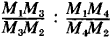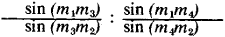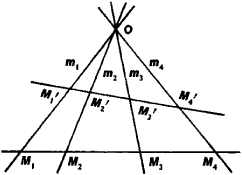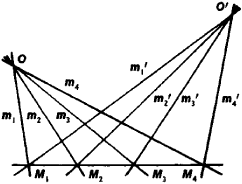# Cross Ratio

(redirected from Cross-ratio)
Also found in: Wikipedia.

## cross ratio

[′krȯs ‚rā·shō]
(mathematics)
For four collinear points, A, B, C, and D, the ratio (AB)(CD)/(AD)(CB), or one of the ratios obtained from this quantity by a permutation of A, B, C, and D.
McGraw-Hill Dictionary of Scientific & Technical Terms, 6E, Copyright © 2003 by The McGraw-Hill Companies, Inc.
The following article is from The Great Soviet Encyclopedia (1979). It might be outdated or ideologically biased.

## Cross Ratio

(also anharmonic ratio). The cross ratio of four collinear points M1M2, M3, M4 (Figure 1) is a number denoted by the symbol (M1M2M3M4 and equal toIn this case, the ratio M1M3/M3M2 is considered to be positive if the direction of the segments M1M3 and M3M2 is the same and negative if the directions are different. The cross ratio depends on the order of the numbering of the points, which may differ from the sequential order of the points on the straight line. In addition to the cross ratio of four points, there is also a cross ratio of four straight lines m1, m2, m3, m4, passing through the point O. This ratio is designated by the symbol (m1m2m3m4) and is equal towhere the angle (mimj) beween the straight lines mi and mj is considered with a sign.Figure 1

If the points M1, M2, M3, M4 lie on the straight lines m1, m2, m3, m4 (Figure 1), then

(M1M2M3m4) = (m1m2m3m3m4)

Hence, if the points M1, M2, M3, M4 and M1’, M2’, M3’, M4’ are the result of the intersection of four straight lines m1, m2, m3, m4 (Figure 1), then (M1M2M3M4’) = (M1M2M3M4). If, however, the straight lines m1, m2, m3, m4 and m1’, m2’, m3’, m4’ project one set of four points M1, M2, Ms, M4 (Figure 2), then (m1m2m3m4’) = (m1m2m3m4).Figure 2

The cross ratio also remains unchanged by any projective transformation, that is, it is an invariant of such transformations, and cross ratios therefore play an important role in projective geometry. Sets of four points or lines for which the cross ratio is equal to 1 are of particular importance. Such sets are called harmonic.

E. G. POZNIAK